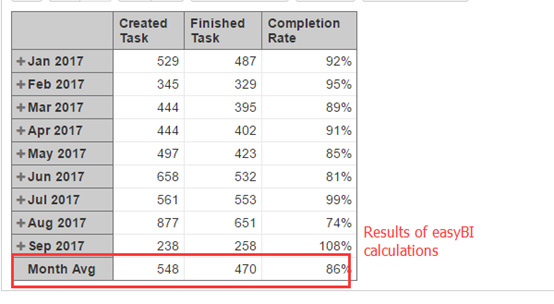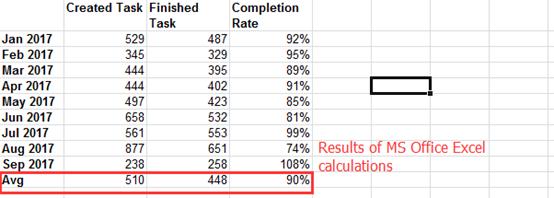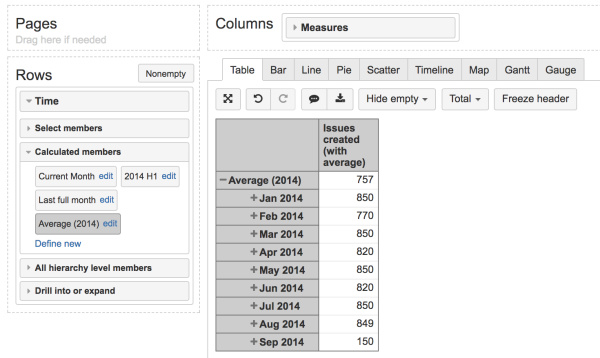# Why is the average result different?

I want to calculate the average of (creation task, the completion of the task, and the completion rate)
The function I wrote is as follows, and the results obtained, Why is it inconsistent with the results calculated in excel?
Month Avg=
Avg([Time].[Month].[Jan 2017]:[Time].[Month].[Sep 2017],[Time].[Month].CurrentMember)Hi,

I would suggest a bit different approach, as the Average does not work in that way. You should add particular measure in the Avg() function to calculate values for a set as the syntax of average is Avg(set, numeric expression); also, this should be done in Measures, not in Time dimension.

1. Create a calculated member in Time dimension containing all months of the year, but without the averge calculation - just for the row where average would be displayed. You can call this calculated member as you want to display it in the report - for instance, “Average (2017)”. The calculation would be the following:

`````` Aggregate({
Filter(
[Time].[Month].Members,
DateInPeriod([Time].CurrentMember.Startdate, [Time].))
})
``````

Select and expand this member in report rows.

2. Then recreate all measures and add case when construction to show the particular measure in the months level, and the average in the Average (2017) row:

``````case when
[Time].CurrentHierarchyMember.Level.Name = "Month"
then